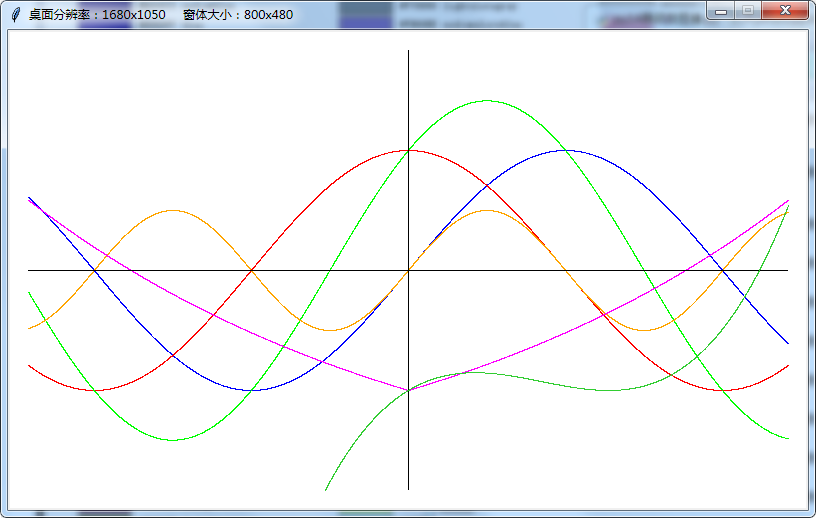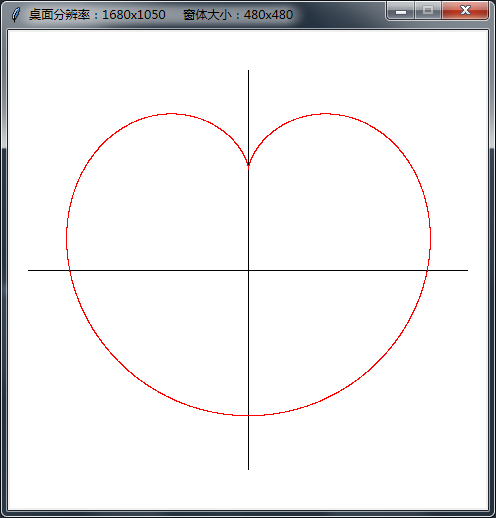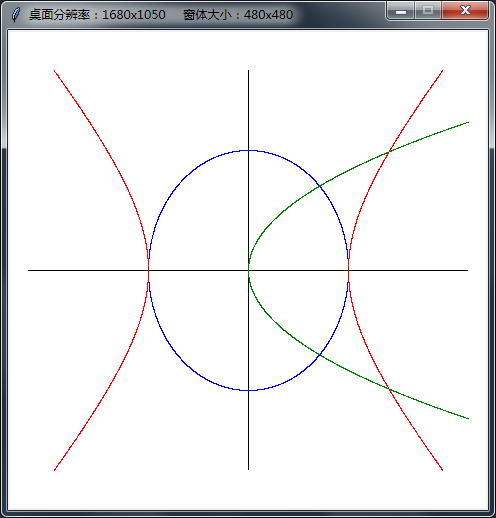• 第2课时 待定系数法求二次函数解析式教学目标能熟练根据已知点坐标的情况，适当的方法求二次函数解析式.教学过程阅读教材第39至40页,自学“探究”，掌握待定系数法求二次函数解析式.自学反馈 学生独立...

第2课时 用待定系数法求二次函数的解析式

教学目标

能熟练根据已知点坐标的情况，用适当的方法求二次函数的解析式.

教学过程

阅读教材第39至40页,自学“探究”，掌握用待定系数法求二次函数的解析式.

自学反馈 学生独立完成后集体订正

①二次函数y=4x2-mx+5，当x-2时，y随x的增大而增大,则当x=1时，y的值为25.

教师讲解 可根据顶点公式用含m的代数式表示对称轴，从而求出m的值.

②抛物线y=-2x2+2x+2的顶点坐标是(,).

③如图所示的抛物线是二次函数y=ax2-3x+a2-1的图象，那么a的值是-1.

教师讲解 可根据图象经过原点求出a的值，再考虑开口方向.

④二次函数y=ax2+bx+c的图象大致位置如图所示，下列判断错误的是( D )

A.a<0 B.b>0 C.c>0 D.b2a>0

第④题图 第⑤题图

⑤如图，抛物线y=ax2+bx+c(a>0)的对称轴是直线x=1，且经过点P(3，0)，则a-b+c的值为( A )

A.0 B.-1 C.1 D.2

教师讲解 根据二次函数图象的对称性得知图象与x轴的另一交点坐标为(-1，0)，将此点代入解析式，即可求出a-b+c的值.

⑥二次函数y=ax2+x+a2-1的图象可能是 ( B )

教师讲解 根据图形确定二次项系数的取值，再找其他特征，直至找到矛盾从而逐一排除.

合作探究

活动1 小组讨论

例1 已知二次函数的图象经过点A(3,0)，B(2，-3)，C(0，-3)，求函数的解析式和对称轴.

解:设函数解析式为y=ax2+bx+c，因为二次函数的图象经过点A(3,0)，B(2，-3)，C(0，-3)，

则有 解得

∴函数的解析式为y=x2-2x-3，其对称轴为直线x=1.

教师讲解 已知二次函数图象经过任意三点，可直接设解析式为一般式，代入可得三元一次方程，解之即可求出待定系数.

例2 已知一抛物线与x轴的交点是A(-2，0)、B(1，0)，且经过点C(2，8).试求该抛物线的解析式及顶点坐标.

解:设解析式为y=a(x+2)(x-1)，则有a(2+2)(2-1)=8,∴a=2.∴此函数的解析式为y=2x2+2x-4，其顶点

坐标为(-，-).

教师讲解 因为已知点为抛物线与x轴的交点，解析式可设为交点式，再把第三点代入可得一元一次方程，较一般式所得的三元一次方程简单.而顶点可根据顶点公式求出.

活动2 课堂训练(学生独立完成后展示学习成果)

1.已知一个二次函数的图象的顶点是(-1,2)，且过点(0,)，求这个二次函数的解析式及与x轴交点的坐标.

解：解析式为y=-x2-x+,与x轴交点坐标为(-3,0)、(1，0).

教师讲解 此题只告诉了两个点的坐标，但其中一点为顶点坐标，所以解析式可设顶点式：y=a(x-h)2+k，即可得到一个关于字母a的一元一次方程，再把另一点代入即可求出待定系数.在设解析式时注意h的符号.关于其图象与x的交点，即当y=0时，解关于x的一元二次方程.

2.若二次函数y=ax2+bx+c的图象过点(1，0)，且关于直线x=对称，那么它的图象还必定经过原点.

3.如图，已知二次函数y=-x2+bx+c的图象经过点A(2，0)，B(0，-6)两点.

①求这个二次函数的解析式；

②设该二次函数的对称轴与x轴交于点C，连接BA、BC，求△ABC的面积.

解：①y=-x2+4x-6； ②6.

教师讲解 ①求解析式一般都用待定系数法；②求底边落在坐标轴上的三角形的面积时第三点纵坐标的绝对值即为三角形的高.

活动3 课堂小结

利用待定系数法求二次函数的解析式，需要根据已知点的情况设适当形式的解析式，可以使解题过程变得更简单.

展开全文• 二次函数解析式的三种形式2020-01-18 10:11:13文/宋则贤一般地，把形如y=ax²+bx+c(a≠0)(a、b、c是常数)的函数叫做二次函数，下面总结了二次函数的表达式，供大家参考。二次函数的三种表达式一般式：y=ax²+bx+c (a...

二次函数解析式的三种形式2020-01-18 10:11:13文/宋则贤

一般地，把形如y=ax²+bx+c(a≠0)(a、b、c是常数)的函数叫做二次函数，下面总结了二次函数的表达式，供大家参考。二次函数的三种表达式

一般式：y=ax²+bx+c (a，b，c为常数，a≠0)

顶点式：y=a(x-h)²+k [抛物线的顶点P(h,k)]

交点式：y=a(x-x₁)(x-x₂) [仅限于与x轴有交点A(x1，0)和B(x2，0)的抛物线]

注意：任何二次函数的解析式都可以化成一般式或顶点式，但并非所有的二次函数都可以写成交点式，只有抛物线与x轴有交点，即b2-4ac≥0时，抛物线的解析式才可以用交点式表示．二次函数解析式的这三种形式可以互化。

二次函数的性质

1.二次函数的图像是抛物线，抛物线是轴对称图形。对称轴为直线x=-b/2a。

2.二次项系数a决定抛物线的开口方向和大小。

3.一次项系数b和二次项系数a共同决定对称轴的位置。

4.常数项c决定抛物线与y轴交点。抛物线与y轴交于(0,c)。

当c>0时，图像与y轴正半轴相交。

当c<0时，图像与y轴负半轴相交。

展开全文• 我们常见的二次函数解析式主要分为：① 一般式；② 顶点式；③ 交点式(两根式)；三种表示形式，针对于一些特殊情况我们可以利用二次函数的另外三种：④对称式法；⑤待定系数法；⑥平移法；来更快的确定出二次函数的...

二次函数在初中数学的知识体系中算得上是一个重要内容， 而在高中数学中只能算得上一个重要的基础知识了，因而起到了一个“承上启下”的作用，所以学好二次函数的相关知识至关重要；我们常见的二次函数解析式主要分为：① 一般式；② 顶点式；③ 交点式(两根式)；三种表示形式，针对于一些特殊情况我们可以利用二次函数的另外三种：④对称式法；⑤待定系数法；⑥平移法；来更快的确定出二次函数的解析式；因而前三种常见的二次函数解析式需要牢记掌握，后三种侧重于方法类，需要灵活进行运用即可.

1.一般式：y=ax²+bx+c(a≠0)

如果已知二次函数的图象上的三个点的坐标(或称函数的三对对应值)(x₁，y₁)、 (x₂，y₂) 、(x₃，y₃)，那么我们可以直接借助方程组：就可以唯一确定a、b、c的值，从而求得函数解析式 y=ax²+bx+c(a≠0)．

总结：      ﻿

①任何二次函数都可以整理成一般式y=ax²+bx+c(a≠0)的形式．

②已知任意3点坐标，可用一般式联立方程组求解二次函数解析式．

2.顶点式：y=a(x－h)²+k (a≠0)

我们可以首先由二次函数的一般式 y=ax²+bx+c(a≠0)进行配方得：总结：

①已知顶点坐标或对称轴时，可用顶点式求解二次函数解析式．

②已知二次函数的顶点和图象上的任意一点，都可以用顶点式来确定解析式．

3.交点式：y=a(x－x₁)(x－x₂) (a≠0)

我们可以根据二次函数的一般式结合配方法推导出二次函数的交点式或者说是两根式：注意：这里 x₁，x₂，分别是一元二次方程ax²+bx+c=0(a≠0)的两个根．

所以针对于题目中已知二次函数的图象与x轴有交点(或者说对应的一元二次方程

ax²+bx+c=0(a≠0)有实根)时，此时我们就可以令函数解析式为y=a(x－x₁)(x－x₂) (a≠0)，从而求得此函数的解析式．

总结：

①已知抛物线与x轴的两个交点坐标，可用交点式求解二次函数解析式．

②已知二次函数与x轴的交点坐标，和图象上任意一点时，可用交点式求解二次函数解析式．

③已知二次函数与x轴的交点坐标(x₁，0)，(x₂，0)，可知二次函数的对称轴为x=( x₁+x₂ )÷2．

④根据二次函数的对称性可知，对于函数图象上的两点(x₁，0)，(x₂，0)，如果它们有相同的纵坐标，则可知二次函数的对称轴为 x=( x₁+x₂ )÷2．

⑤同时针对于任意的二次函数 y=ax²+bx+c(a≠0)我们可以进行如下推导：4.对称式： y=a(x－x₁)(x－x₂) +k (a≠0)

总结：

当抛物线经过点(x₁，k)，(x₂，k) 时，我们可以直接设出对称式：

y=a(x－x₁)(x－x₂) +k (a≠0)，然后再将另一个坐标代入式子中，求出a的值即可确定二次函数的解析式．

注意：

任何二次函数的解析式都可以化成一般式或顶点式，但并非所有的二次函数都可以写成交点式，只有抛物线与轴有交点，即b²-4ac≥0时，抛物线的解析式才可以用交点式表示，同时要注意任意二次函数的解析式这三种基本形式都是可以互化的．

5.待定系数法确定解析式6.平移法确定解析式

(1)化成顶点式后平移：

①先利用配方法把二次函数化成 y=a(x－h)²+k (a≠0) 的形式，接着再利用顶点的平移来确定新的顶点坐标，然后再写出新的函数解析式即可．

②最后在原有函数的基础遵从“左加右减，上加下减”的规则进行平移即可解答．

(2)对一般式直接平移：

对于任意的二次函数y=ax²+bx+c(a≠0)的平移，也都可以直接用“左加右减，上加下减”来进行平移．

本文由Math实验室原创文章，如果你需要可编辑的文档文件可以联系我，希望本文对你有所帮助~

展开全文c语言判断二次函数
• Canvas没有画点的函数，我自定义函数Graph()某一点到其右下相邻点的线段来代替。然后把给定函数f(x)在指定区间里的点 (x, f(x)) 依次画出，步进间隔小到一定程度就会连成f(x)对应的曲线。 for ... in range()的...

Canvas没有画点的函数，我们就用某一点到其右下相邻点的连线来代替。然后自定义一个函数Graph()，把给定函数f(x)在指定区间里的点 (x, f(x)) 依次画出，步进间隔小到一定程度就会连成f(x)对应的曲线。

for ... in range()的步进值只能是整数，所以引入numpy库的arange()，其步进可以是小数，例： for i in numpy.arange(0,2,0.01) # 表示区间[0,1)上步进0.01，共循环200次。

函数解析式的图像

import tkinter as tk
import pyautogui as ag
from math import *
from numpy import arange as np

def Window_Open(W, H):
X, Y = ag.size()
winSize = str(W)+"x"+str(H)
winPos = winSize + "+" + str((X - W) // 2)
winPos += "+" + str((Y - H) // 2)
win.geometry(winPos)
win.resizable(False, False)
title = u'桌面分辨率：' + str(X) + "x" + str(Y)
title += ' ' * 5 + u'窗体大小：' + winSize
win.title(title)
win.update()

def Graph(func,x0,y0,xmin,xmax,w,h,c='blue',step=0.001):
'xmin,xmax 自变量的取值范围； c 图像颜色'
'x0,y0 原点坐标  w,h 横纵轴半长 step 步进'
coord = x0-w,y0,x0+w,y0
tCanvas.create_line(coord,fill='black')
coord = x0,y0-h,x0,y0+h
tCanvas.create_line(coord,fill='black')
w1,w2=100,120 # w1,w2为自变量和函数值在横纵轴上的放大倍数
for x in np(xmin,xmax+step,step):
y = func(x)
coord = x0+w1*x,y0-w2*y,x0+w1*x+1,y0-w2*y+1
if abs(x*w1)<w and abs(y*w2)<h:
tCanvas.create_line(coord,fill=c)
tCanvas.update()

if __name__ == '__main__':

win = tk.Tk()
Window_Open(800,480)
tCanvas = tk.Canvas(win, width=win.winfo_width(), height=480, bg='white')
tCanvas.pack(side="top")

x0,y0=400,240   # 原点坐标
xmax=pi*2       # 自变量最大值，超过横坐标最大值被截短
w,h=380,220     # 横纵轴的正向最大值

fx1 = lambda x : sin(x)+cos(x)
fx2 = lambda x : sin(x)*cos(x)
gx1 = lambda x : exp(abs(x/4))-2
gx2 = lambda x : (x/2)**3-2*(x/2)**2+x/2-1

Graph(sin,x0,y0,-xmax,xmax,w,h)
Graph(cos,x0,y0,-xmax,xmax,w,h,'red')
Graph(fx1,x0,y0,-xmax,xmax,w,h,'lime')
Graph(fx2,x0,y0,-xmax,xmax,w,h,'orange')
Graph(gx1,x0,y0,-xmax,xmax,w,h,'magenta')
Graph(gx2,x0,y0,-xmax,xmax,w,h,'limegreen')

win.mainloop()

效果图：心形曲线参数方程的图像

把Graph()函数稍作改进，就能画出参数方程对应的曲线图像，如下例：心形曲线的图像

import tkinter as tk
import pyautogui as ag
from math import *
from numpy import arange as np

def Window_Open(W, H):
X, Y = ag.size()
winSize = str(W)+"x"+str(H)
winPos = winSize + "+" + str((X - W) // 2)
winPos += "+" + str((Y - H) // 2)
win.geometry(winPos)
win.resizable(False, False)
title = u'桌面分辨率：' + str(X) + "x" + str(Y)
title += ' ' * 5 + u'窗体大小：' + winSize
win.title(title)
win.update()

def Graph(funcx,funcy,x0,y0,tmin,tmax,w,h,c='blue',step=0.001):
'xmin,xmax 自变量的取值范围； c 图像颜色'
'x0,y0 原点坐标  w,h 横纵轴半长 step 步进'
coord = x0-w,y0,x0+w,y0
tCanvas.create_line(coord,fill='black')
coord = x0,y0-h,x0,y0+h
tCanvas.create_line(coord,fill='black')
w1,w2=100,110 # w1,w2为自变量和函数值在横纵轴上的放大倍数
for t in np(tmin,tmax+step,step):
x = funcx(t)
y = funcy(t)
coord = x0+w1*x,y0-w2*y,x0+w1*x+1,y0-w2*y+1
if abs(x*w1)<w and abs(y*w2)<h:
tCanvas.create_line(coord,fill=c)
tCanvas.update()

if __name__ == '__main__':

win = tk.Tk()
Window_Open(480,480)
tCanvas = tk.Canvas(win, width=win.winfo_width(), height=480, bg='white')
tCanvas.pack(side="top")

x0,y0=240,240   # 原点坐标

a = 0.7

fx = lambda t : a*(2.0*sin(t)-sin(2*t))
fy = lambda t : a*(1.6*cos(t)-cos(2*t))+0.5

Graph(fx,fy,x0,y0,-pi,pi,220,200,c='red')

win.mainloop()

效果图：椭圆、双曲线、抛物线的图像

import tkinter as tk
import pyautogui as ag
from math import *
from numpy import arange as np

def Window_Open(W, H):
X, Y = ag.size()
winSize = str(W)+"x"+str(H)
winPos = winSize + "+" + str((X - W) // 2)
winPos += "+" + str((Y - H) // 2)
win.geometry(winPos)
win.resizable(False, False)
title = u'桌面分辨率：' + str(X) + "x" + str(Y)
title += ' ' * 5 + u'窗体大小：' + winSize
win.title(title)
win.update()

def Graph(funcx,funcy,x0,y0,tmin,tmax,w,h,c='blue',step=0.001):
'xmin,xmax 自变量的取值范围； c 图像颜色'
'x0,y0 原点坐标  w,h 横纵轴半长 step 步进'
coord = x0-w,y0,x0+w,y0
tCanvas.create_line(coord,fill='black')
coord = x0,y0-h,x0,y0+h
tCanvas.create_line(coord,fill='black')
w1,w2=100,100 # w1,w2为自变量和函数值在横纵轴上的放大倍数
for t in np(tmin,tmax+step,step):
x = funcx(t)
y = funcy(t)
coord = x0+w1*x,y0-w2*y,x0+w1*x+1,y0-w2*y+1
if abs(x*w1)<w and abs(y*w2)<h:
tCanvas.create_line(coord,fill=c)
tCanvas.update()

if __name__ == '__main__':

win = tk.Tk()
Window_Open(480,480)
tCanvas = tk.Canvas(win, width=win.winfo_width(), height=480, bg='white')
tCanvas.pack(side="top")

x0,y0=240,240
a,b = 1,1.2
p = 0.5

f1x = lambda t : a*cos(t)
f1y = lambda t : b*sin(t)

f2x = lambda t : a/cos(t)
f2y = lambda t : b*tan(t)

f3x = lambda t : 2*p*t**2
f3y = lambda t : 2*p*t

Graph(f1x,f1y,x0,y0,-pi,pi,220,200)
Graph(f2x,f2y,x0,y0,-pi,pi,220,200,c='red')
Graph(f3x,f3y,x0,y0,-pi,pi,220,200,c='green')

win.mainloop()

效果图：附：圆锥曲线的参数方程

椭圆
x = a*cost
y = b*sint
双曲线
x = a*sect
y = b*tant
抛物线
x = 2*p*t²
y = 2*p*t

展开全文python tkinter Canvas 参数方程
• 而im2double是将图像数据double转换后，做了归一化处理 【imfilter，fspecial与imresize】 out=imfilter(in,fspecial('gaussian',7,1),'same','replicate') %25表示你产生的滤波器的大小是7×7的。1是说你的高斯...
• 其实在java8就已经有java的函数式编程写法，只是难度较大，大家习惯了对象用法，但在其它语言中都有函数式的用法，如js,scala，函数式其实是抽象到极致的思想。什么是函数式编程函数式编程并不是Java新提出的...java 函数式编程
• Python函数解析

2021-09-18 15:37:53
函数就是组织好的、可重复使用的，并实现相关功能的代码段,使用函数能够提高代码的重复使用率、也减少了代码量，提高了代码的可维护性。比如Python中的print()就是一个函数，只不过print()函数是Python自带的，我们...python pycharm flask
• 回答：lnx表示成log(x) 而lgx表示成log10(x)话题：怎样MATLAB语言表示ln(x),log3(x),arcsin(x)还有x开6次方呀回答：log(x)asin(x)x^-6话题：#在matlab中怎样使用回答：punct-Functionhandlecreation# #在匿...
• 文章目录前言函数式接口介绍@FunctionalInterface注解函数式接口的使用常用函数式接口Supplier接口抽象方法getConsumer接口介绍抽象方法accept默认方法andThenPredicate接口介绍抽象方法test默认方法and默认方法or...java 函数式编程 lambda
• 列表解析式也称之为列表推导式，即List Comprehensions，是Python内置的非常简单却强大的可以用来创建列表的生成式。本质上就是列表来构建列表，通过对已有列表中的每一项应用一个指定的表达式来构建出一个新的...
• 心形函数的几种表达式

万次阅读 2021-08-25 00:03:17
两个函数表示： f(x)=sqrt(1-(abs(x)-1)^2) h(x)=-2*sqrt(1-0.5*abs(x)) 也可以根据图中的q(x)画出心形的内部： q(x)=(f(x)-h(x))/2*cos(200*x)+(f(x)+h(x))/2 一个函数表示，我拟合了很久才画出来的： ...
• 七夕节是个浪漫的节日，利用几何画板这个强大的绘图软件也可以对心爱的人表示！笛卡尔心形线像极了人的一颗心，用来表达爱意再好不过了。本文我们来介绍具体步骤如下：1.新建参数。右键绘图区空白处，“新建参数”，...
• dsolve函数是matlab中自带的可以用来解决部分常微分方程的函数(方法)，下面以物理学中的热传导问题为例展示如何使用这个函数。热力学模型假设物体和恒温的环境接触，温度随时间的变化率和温差成正比，我们假设环境...
• python的几个魔法函数__repr__Python中这个__repr__函数，对应repr(object)这个函数，返回一个可以用来表示对象的可打印字符串.如果我们直接打印一个类，向下面这样class A():def __init__(self,name=None,id=1):...
• Java 8 函数式编程本次主题主要介绍什么是函数式编程,其主要特点, 以及它在 Java8 中是怎么体现的.函数式编程这个思维早在1958年就被提出来的了,在60年后的今天重新被提出来,且越来越流行,其主要原因是 CPU 的时钟...
• 复变函数（2）-复变函数及其解析性                            东风夜放花千树，更吹落，星如雨       2.1 复变函数的定义：  设DDD是复平面上一个非空点集。如果...
• 分段函数是初等函数吗

千次阅读 2020-12-18 22:19:49
2019-10-09阅读(61)金圣叹先生传①先生金姓，采名，若采字，吴县诸生也。...每升座开讲，声音宏亮2019-10-09阅读(77)富字组词有：富寿(富寿)[fùshòu]孔子家语·贤君》：“政之急者，莫大乎使民富且寿也。...
• 函数模板是通用的函数描述，它们使用泛型来定义函数，其中的泛型可用具体的类型替换。 通过将类型作为参数传递给模板，可使编译器生成该类型的函数。 由于模板允许以泛型（而不是具体类型）的方式编写程序，因此有...c++
• 函数图像

2021-06-28 13:04:47
在数学中，函数 f 的图形(或图象)指的是所有有序对(x, f(x))组成的集合。具体而言，如果x为实数，则函数图形在平面直角坐标系上呈现为一条曲线。如果函数自变量x为两个实数组成的有序对(x1, x2)，则图形就是所有...
• 要不要使用复杂表达式Perl语言的原作者Larry Wall曾经说过，伟大的程序员有三个优点：懒惰、暴躁和自负。乍一看这三个词语没有一个是褒义词，但在程序员的世界里，这三个词有不同的意义。首先，懒惰会促使程序员去...
• 复变函数项级数2.1 基本概念 1. 复数项级数 1.1 复数序列 定义 设znz_nzn​为复数，称{zn}n=1,2,⋯\{z_n \}_{n=1,2,\cdots}{zn​}n=1,2,⋯​为复数序列 极限 存在自然数N，使得当n > N时，总有∣zn−α∣<ϵ|...数学 数字通信
• 《计算机二级常用函数公式及解析》由会员分享，可在线阅读，更多相关《计算机二级常用函数公式及解析(9页珍藏版)》请在人人文库网上搜索。1、1、求和函数SUM 功能：将参数中的所有数字相加求和；其中每个参数既可以...
• 二次函数的顶点坐标是(h，k)，将其代入顶点式y=a(x-h)²+...2、顶点式：y=a(x-h)²+k(a≠0，a、h、k为常数)3、交点式(与x轴)：y=a(x-x1)(x-x2)(a≠0，x1、x2为常数)待定系数法求二次函数解析式1、当题给条件为已...
• 1、BigInteger(byte[] val)这个构造...(字节数组中值的ASCII码构造BigInteger)2、BigInteger(int signum, byte[] magnitude)此构造函数用于将BigInteger的符号大小表示法转换成一个BigInteger值。(和第一种一样，...
• 提到数学你会想到什么？...从今天开始中考君将给您讲述不一样的数学世界，从不同的角度解析数学大佬怎么玩转数学。第一篇就从“勒内·笛卡尔”说起。笛卡尔坐标系的创始人，现代哲学思想的奠基人，...
• 二次函数顶点、交点、两根考查一定是初中阶段平时考试和中考的重要考点，所以秦学教育小编这里再一次对二次函数顶点、交点、两根概念进行一个解读。一般地，自变量x和因变量y之间存在如下关系：(1)一般...抛物线交点式公式
• 全文共2568字，预计学习时长5分钟或更长图片来源：Pixabay/Gerd Altmann本篇文章将详细介绍解析式的基本要素及其各种形式。Python中的解析式解析式是允许在其他序列中构建序列的结构。Python 2.0介绍了列表解析式的...
• 在调用函数的过程中参数是如何被解析先看第一个问题，在python中函数参数的定义主要有四种方式：1.F(arg1,arg2,...)这是最常见的定义方式，一个函数可以定义任意个参数，每个参数间逗号分割，这种方式定义的函数...python参数讲解
• 数学表白函数—心形线

万次阅读 2020-12-19 13:38:06
plot(x,y) 此外，还可仪逐点生成算法实现 其实心形的函数线不仅仅只有上述的一种如： 在几何画板中画出 还有 看到这些你是否有些小激动，快拿起数学的“武器”向你的女神男神表出心意吧（当然你的那个她未必看得...爱心的数学函数方程...